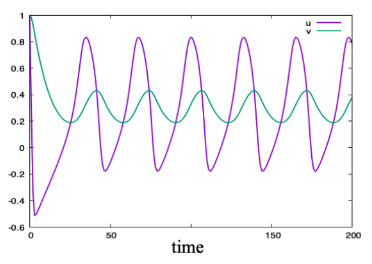# FitzHugh–Nagumo ODEs

##### Persistent Identifier

ODEs for the FitzHugh–Nagumo well-mixed system

## Introduction

The FitzHugh–Nagumo (FN) model is commonly used as a toy model for neuronal excitation.

## Description

Everything is well-mixed and two ODEs are solved for two variables, $u$ (voltage) and $v$ (recovery variable).

## Results

The figure below shows behavior of the FitzHugh–Nagumo ODEs, the variables $u$, $v$ are plotted against time.Variables $u$, $v$ plotted against time.

## Model

Get this model via:

•  Download: FN.xml
• XML Preview

<MorpheusModel version="4">
<Description>
<Title>FitzHugh–Nagumo ODEs</Title>
<Details>Full title:		FitzHugh–Nagumo ODEs
Authors:		L. Edelstein-Keshet
Contributors:	Y. Xiao
Date:		10.05.2022
Model ID:		https://identifiers.org/morpheus/M2005
Reference:		L. Edelstein-Keshet: Mathematical Models in Cell Biology
Comment:		ODEs for the FN well-mixed system. The FN equations are commonly used as a toy model for neuronal excitation. u = "voltage", v = "recovery variables". Everything is well-mixed and two ODEs are solved for two variables, u and v.</Details>
</Description>
<Global>
<Variable symbol="u" name="Voltage" value="1"/>
<Variable symbol="v" name="Recovery Variable" value="1"/>
<System time-step="0.1" solver="Runge-Kutta [fixed, O(4)]">
<Constant symbol="a" name="middle value" value="0.25"/>
<Constant symbol="b" name="v decay rate" value="1.0"/>
<Constant symbol="epsilon" name="slow relative timescale" value="0.05"/>
<Constant symbol="I_0" name="Stimulus" value="0.25"/>
<DiffEqn symbol-ref="u">
<Expression> u*(1-u)*(u-a)-v + I_0  </Expression>
</DiffEqn>
<DiffEqn symbol-ref="v">
<Expression> epsilon*(u-b*v)</Expression>
</DiffEqn>
</System>
</Global>
<Space>
<Lattice class="linear">
<Size symbol="size" value="1, 0, 0"/>
<Neighborhood>
<Order>1</Order>
</Neighborhood>
</Lattice>
<SpaceSymbol symbol="space"/>
</Space>
<Time>
<StartTime value="0"/>
<StopTime symbol="stoptime" value="200"/>
<TimeSymbol symbol="time"/>
</Time>
<Analysis>
<Logger time-step="1">
<Input>
<Symbol symbol-ref="u"/>
<Symbol symbol-ref="v"/>
</Input>
<Plots>
<Plot time-step="-1">
<Style line-width="2.0" style="lines"/>
<Terminal terminal="png"/>
<X-axis>
<Symbol symbol-ref="time"/>
</X-axis>
<Y-axis>
<Symbol symbol-ref="u"/>
<Symbol symbol-ref="v"/>
</Y-axis>
</Plot>
</Plots>
<Output>
<TextOutput/>
</Output>
</Logger>
<ModelGraph format="svg" reduced="false" include-tags="#untagged"/>
</Analysis>
</MorpheusModel>